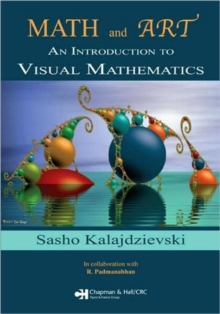Supporting your high street Find out how »
• My Account# Math and Art : An Introduction to Visual Mathematics Paperback / softback

#### Description

Math and Art: An Introduction to Visual Mathematics explores the potential of mathematics to generate visually appealing objects and reveals some of the beauty of mathematics.

With a CD-ROM and a 16-page full-color insert, it includes numerous illustrations, computer-generated graphics, photographs, and art reproductions to demonstrate how mathematics can inspire art.

Basic Math Topics and Their Visual Aspects Focusing on accessible, visually interesting, and mathematically relevant topics, the text unifies mathematics subjects through their visual and conceptual beauty.

Sequentially organized according to mathematical maturity level, each chapter covers a cross section of mathematics, from fundamental Euclidean geometry, tilings, and fractals to hyperbolic geometry, platonic solids, and topology.

For art students, the book stresses an understanding of the mathematical background of relatively complicated yet intriguing visual objects.

For science students, it presents various elegant mathematical theories and notions.

Comprehensive Material for a Math in Art Course Providing all of the material for a complete one-semester course on mathematics in art, this self-contained text shows how artistic practice with mathematics and a comprehension of mathematical concepts are needed to logically and creatively appreciate the field of mathematics.

#### Information

• Format: Paperback / softback
• Pages: 272 pages, 16 page color insert follows pg.144; 21 equations; 20 Halftones, black and white; 1 Table
• Publisher: Taylor & Francis Inc
• Publication Date:
• Category: General arts
• ISBN: 9781584889137

£46.99

£40.95

on all orders

###### Pick up orders

from local bookshops